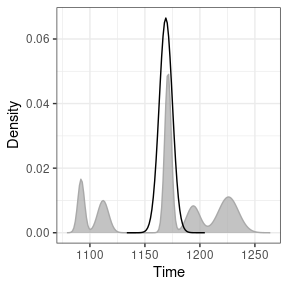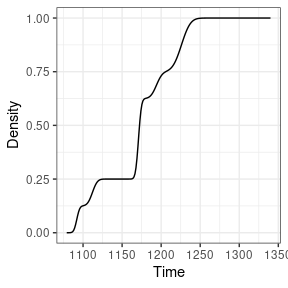# Event Date Model

#### 2022-11-08

# Load packages
library(folio) # Datasets
library(kairos)

## Definition

Event and accumulation dates are density estimates of the occupation and duration of an archaeological site (L. Bellanger, Husi, and Tomassone 2006; L. Bellanger, Tomassone, and Husi 2008; Lise Bellanger and Husi 2012).

The event date is an estimation of the terminus post-quem of an archaeological assemblage. The accumulation date represents the “chronological profile” of the assemblage. According to Lise Bellanger and Husi (2012), accumulation date can be interpreted “at best […] as a formation process reflecting the duration or succession of events on the scale of archaeological time, and at worst, as imprecise dating due to contamination of the context by residual or intrusive material.” In other words, accumulation dates estimate occurrence of archaeological events and rhythms of the long term.

### Event Date

Event dates are estimated by fitting a Gaussian multiple linear regression model on the factors resulting from a correspondence analysis - somewhat similar to the idea introduced by Poblome and Groenen (2003). This model results from the known dates of a selection of reliable contexts and allows to predict the event dates of the remaining assemblages.

First, a correspondence analysis (CA) is carried out to summarize the information in the count matrix $$X$$. The correspondence analysis of $$X$$ provides the coordinates of the $$m$$ rows along the $$q$$ factorial components, denoted $$f_{ik} ~\forall i \in \left[ 1,m \right], k \in \left[ 1,q \right]$$.

Then, assuming that $$n$$ assemblages are reliably dated by another source, a Gaussian multiple linear regression model is fitted on the factorial components for the $$n$$ dated assemblages:

$t^E_i = \beta_{0} + \sum_{k = 1}^{q} \beta_{k} f_{ik} + \epsilon_i ~\forall i \in [1,n]$ where $$t^E_i$$ is the known date point estimate of the $$i$$th assemblage, $$\beta_k$$ are the regression coefficients and $$\epsilon_i$$ are normally, identically and independently distributed random variables, $$\epsilon_i \sim \mathcal{N}(0,\sigma^2)$$.

These $$n$$ equations are stacked together and written in matrix notation as

$t^E = F \beta + \epsilon$

where $$\epsilon \sim \mathcal{N}_{n}(0,\sigma^2 I_{n})$$, $$\beta = \left[ \beta_0 \cdots \beta_q \right]' \in \mathbb{R}^{q+1}$$ and

$F = \begin{bmatrix} 1 & f_{11} & \cdots & f_{1q} \\ 1 & f_{21} & \cdots & f_{2q} \\ \vdots & \vdots & \ddots & \vdots \\ 1 & f_{n1} & \cdots & f_{nq} \end{bmatrix}$

Assuming that $$F'F$$ is nonsingular, the ordinary least squares estimator of the unknown parameter vector $$\beta$$ is:

$\widehat{\beta} = \left( F'F \right)^{-1} F' t^E$

Finally, for a given vector of CA coordinates $$f_i$$, the predicted event date of an assemblage $$t^E_i$$ is:

$\widehat{t^E_i} = f_i \hat{\beta}$

The endpoints of the $$100(1 − \alpha)$$% associated prediction confidence interval are given as:

$\widehat{t^E_i} \pm t_{\alpha/2,n-q-1} \sqrt{\widehat{V}}$

where $$\widehat{V_i}$$ is an estimator of the variance of the prediction error: $\widehat{V_i} = \widehat{\sigma}^2 \left( f_i^T \left( F'F \right)^{-1} f_i + 1 \right)$

were $$\widehat{\sigma} = \frac{\sum_{i=1}^{n} \left( t_i - \widehat{t^E_i} \right)^2}{n - q - 1}$$.

The probability density of an event date $$t^E_i$$ can be described as a normal distribution:

$t^E_i \sim \mathcal{N}(\widehat{t^E_i},\widehat{V_i})$

### Accumulation Date

As row (assemblages) and columns (types) CA coordinates are linked together through the so-called transition formulae, event dates for each type $$t^E_j$$ can be predicted following the same procedure as above.

Then, the accumulation date $$t^A_i$$ is defined as the weighted mean of the event date of the ceramic types found in a given assemblage. The weights are the conditional frequencies of the respective types in the assemblage (akin to the MCD).

The accumulation date is estimated as: $\widehat{t^A_i} = \sum_{j = 1}^{p} \widehat{t^E_j} \times \frac{x_{ij}}{x_{i \cdot}}$

The probability density of an accumulation date $$t^A_i$$ can be described as a Gaussian mixture:

$t^A_i \sim \frac{x_{ij}}{x_{i \cdot}} \mathcal{N}(\widehat{t^E_j},\widehat{V_j}^2)$

Interestingly, the integral of the accumulation date offers an estimates of the cumulative occurrence of archaeological events, which is close enough to the definition of the tempo plot introduced by Dye (2016).

## Limitation

Event and accumulation dates estimation relies on the same conditions and assumptions as the matrix seriation problem. Dunnell (1970) summarizes these conditions and assumptions as follows.

The homogeneity conditions state that all the groups included in a seriation must:

• Be of comparable duration.
• Belong to the same cultural tradition.
• Come from the same local area.

The mathematical assumptions state that the distribution of any historical or temporal class:

• Is continuous through time.
• Exhibits the form of a unimodal curve.

Theses assumptions create a distributional model and ordering is accomplished by arranging the matrix so that the class distributions approximate the required pattern. The resulting order is inferred to be chronological.

Predicted dates have to be interpreted with care: these dates are highly dependent on the range of the known dates and the fit of the regression.

## Usage

This package provides an implementation of the chronological modeling method developed by Lise Bellanger and Husi (2012). This method is slightly modified here and allows the construction of different probability density curves of archaeological assemblage dates (event, activity and tempo).

## Bellanger et al. did not publish the data supporting their demonstration:
## no replication of their results is possible.
## Here is a pseudo-reproduction using the zuni dataset

## Assume that some assemblages are reliably dated (this is NOT a real example)
## The names of the vector entries must match the names of the assemblages
zuni_dates <- c(
LZ0569 = 1097, LZ0279 = 1119, CS16 = 1328, LZ0066 = 1111,
LZ0852 = 1216, LZ1209 = 1251, CS144 = 1262, LZ0563 = 1206,
LZ0329 = 1076, LZ0005Q = 859, LZ0322 = 1109, LZ0067 = 863,
LZ0578 = 1180, LZ0227 = 1104, LZ0610 = 1074
)

## Model the event and accumulation date for each assemblage
model <- event(zuni, dates = zuni_dates, cutoff = 90)
summary(get_model(model))
#>
#> Call:
#> stats::lm(formula = date ~ ., data = data)
#>
#> Residuals:
#>  LZ0569  LZ0279    CS16  LZ0066  LZ0852  LZ1209   CS144  LZ0563  LZ0329 LZ0005Q
#>  0.4466 -3.9079 -0.2887  0.7279 -1.3127  0.5037  2.7819 -4.4530 -0.7960  0.1254
#>  LZ0322  LZ0067  LZ0578  LZ0227  LZ0610
#>  2.6011 -0.1375  3.5140 -0.7672  0.9624
#>
#> Coefficients:
#>              Estimate Std. Error t value Pr(>|t|)
#> (Intercept) 1164.6501     2.9154 399.481 2.36e-10 ***
#> F1          -158.2879     1.6497 -95.948 7.07e-08 ***
#> F2            25.7034     1.6841  15.263 0.000107 ***
#> F3            -5.6058     2.1603  -2.595 0.060368 .
#> F4            10.7571     5.8231   1.847 0.138419
#> F5            -3.1388     3.9485  -0.795 0.471160
#> F6             2.7244     1.3288   2.050 0.109653
#> F7             4.5647     5.2198   0.874 0.431212
#> F8            11.2980     3.4629   3.263 0.031006 *
#> F9            -5.1715     2.9554  -1.750 0.155041
#> F10           -0.3957     2.6497  -0.149 0.888522
#> ---
#> Signif. codes:  0 '***' 0.001 '**' 0.01 '*' 0.05 '.' 0.1 ' ' 1
#>
#> Residual standard error: 4.09 on 4 degrees of freedom
#> Multiple R-squared:  0.9997, Adjusted R-squared:  0.999
#> F-statistic:  1433 on 10 and 4 DF,  p-value: 1.167e-06

## Estimate event dates
event <- predict_event(model, margin = 1, level = 0.95)
#>        date lower upper error
#> LZ1105 1169  1152  1185     6
#> LZ1103 1143  1136  1150     2
#> LZ1100 1156  1141  1172     5
#> LZ1099 1099  1087  1111     4
#> LZ1097 1088  1078  1099     4
#> LZ1096  840   825   855     5

## Estimate accumulation dates
acc <- predict_accumulation(model)
#> LZ1105 LZ1103 LZ1100 LZ1099 LZ1097 LZ1096
#>   1170   1140   1158   1087   1092    875
## Activity plot
plot(model, type = "activity", event = TRUE, select = "LZ1105")## Tempo plot
plot(model, type = "tempo", select = "LZ1105")Resampling methods can be used to check the stability of the resulting model. If jackknife() is used, one type/fabric is removed at a time and all statistics are recalculated. In this way, one can assess whether certain type/fabric has a substantial influence on the date estimate. If bootstrap() is used, a large number of new bootstrap assemblages is created, with the same sample size, by resampling the original assemblage with replacement. Then, examination of the bootstrap statistics makes it possible to pinpoint assemblages that require further investigation.

## Check model variability
## Warning: this may take a few seconds

## Jackknife fabrics
jack <- jackknife(model)
#>        date lower upper error bias
#> LZ1105 1169  1153  1186     6    0
#> LZ1103 1143  1137  1150     2    0
#> LZ1100 1158  1142  1173     5   34
#> LZ1099 1097  1085  1109     4  -34
#> LZ1097 1094  1083  1104     4  102
#> LZ1096  842   827   857     5   34

## Bootstrap of assemblages
boot <- bootstrap(model, n = 30)
#> LZ1096  726  844.600 1124  726.00  993.05